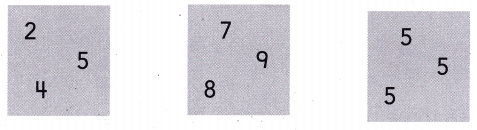Refer to our Texas Go Math Grade 1 Answer Key Pdf to score good marks in the exams. Test yourself by practicing the problems from Texas Go Math Grade 1 Lesson 12.2 Answer Key Add 3 Numbers.

Explore

Use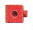to model the problem. Draw to show your work.
___________ birds

FOR THE TEACHER • Read the following problem. Kelly sees 7 birds. Bill sees 2 birds. Joe sees 3 birds. How many birds do they see?
Given,
As Kelly sees seven birds,
Bill sees two birds,
Joe sees three birds,
So by adding seven, two and three we get twelve which means there are twelve birds.

Math Talk
Mathematical Processes

Which two addends did you odd first? Explain.

Model and Draw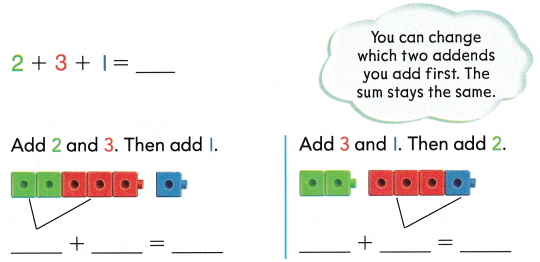Given,
There are three addends in the given number sentence,
At first by adding two with three and then adding one we get the sum as six,
Next one as by adding three with one and then adding two we get the sum as six,
Even though changing the addends positions we get the sum as the same.

Share and Show

Useto change which two addends you add first. Complete the addition sentences.

Question 1.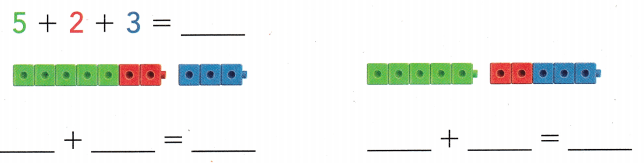Given,
By adding five with two and then adding three we get the sum as ten,
In the same way, we can change the addends and get the sum as the same,
By adding two and three we get five and then adding five with five we get the sum as ten.

Question 2.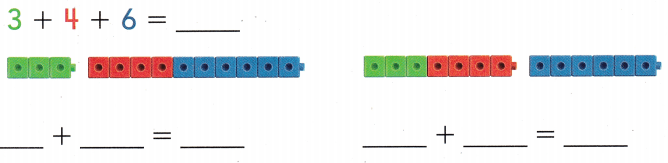Given,
By adding four with six we get ten and then ten with three we get the sum as thirteen,
In the same way we can change the addends to get the same sum,
By adding three with four we get seven and then by adding seven with six we get thirteen as the sum.

Problem Solving

Look at the. Complete the addition sentences showing two ways to find the sum.

Question 3.Given,
By adding three with one we get four, then by adding four with seven we get the sum as eleven,
In the same way, we can change the addends even though we get the same sum,
So by adding seven with three we get ten and then by adding ten with one we get the sum as eleven.

Question 4.Given,
By adding three with three we get six, and then adding six with six we get twelve as the sum,
In the same way, we can change the addends even though we can get the sum as the same,
By adding six with three we get the sum as nine, and then adding nine with three we get the sum as twelve.

Question 5.
H.O.T. I usedto model 3 addends. Use my model. Write the 3 addends.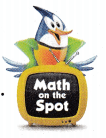My Model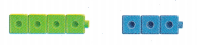_____ + _______ + _________ = 7
Answer: 2 + 2 + 3 = 7

Explanation:
Given,
By adding two with two we get four, and then adding four with three we get seven as the sum,
We can get the sum by adding two addends and even with three addends, even though there is change in the addends the sum remains the same.

Question 6.
Without adding, circle the group of numbers that you think will have the greater sum.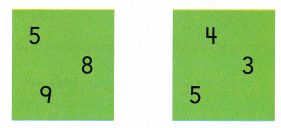How did you estimate?
We can circle the group of numbers as eight and nine as by adding wight with nine we get the greater sum of seventeen,
In the same way, the second group can be four and five as by adding four with five we get the greater sum of nine.

Use. Choose the correct answer.

Question 7.
There are 7 camels in the desert. There are 5 camels on the hill. There are 3 camels by the water. How many camels are there?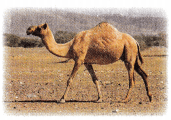(A) 17
(B) 15
(C) 10
Given,
There are seven camels in the desert, five camels on the hill and three camels by the water.
So by adding seven with five we get twelve and then adding twelve with three we get fifteen.
So the answer is there are fifteen camels.

Question 8.
Apply There are 6 people wearing jeans. There are 2 people wearing dresses. There are 4 people wearing shorts. How many people are there?
(A) 12
(B) 8
(C) 17
Given,
There are six people wearing jeans, two people wearing dresses and four people wearing shorts,
So by adding six with two we get eight and adding eight with four we get twelve as the sum,
So there are twelve people.

Question 9.
Multi-Step There are 6 books on the shelf. Julie puts 4 books on the shelf. Michelle puts 5 books on the shelf. Then Julie puts 3 more books on the shelf. How many books are on the shelf?(A) 10
(B) 19
(C) 18
Given,
There are six books on the shelf,
Julie puts four books on the shelf,
Michelle has five books on the shelf,
Julie puts three more books on the shelf.
So by adding six with four we get ten,
then by adding ten with five we get fifteen,
by adding fifteen with three we get eighteen.
So the answer is there are eighteen books on the shelf.

Question 10.
Texas Test Prep What is the sum for 2 + 2 + 8?
(A) 20
(B) 11
(C) 12
Given,
By adding two with two we get four
and then adding four with eight we get twelve as the sum,

TAKE HOME ACTIVITY • Have your child draw to show two ways to odd the numbers 2, 4, and 6.

### Texas Go Math Grade 1 Lesson 12.2 Homework and Practice Answer Key

Look at theto change which addends you add first. Complete the addition sentences.

Question 1.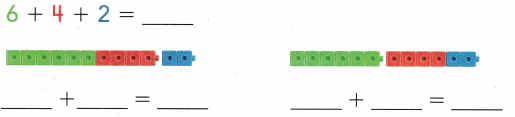Given,
By adding six with four we get ten,
and then by adding ten with two we get twelve as the sum,
In the same way we change the addends and get the same sum,
So by adding four with two we get six and then six with six we get twelve as the sum.
Even though there is change in addends, the sum remains the same.

Question 2.Given,
By adding six with one we get seven,
And then by adding seven with two we get nine as the sum,
In the same way we can switch the addends and the get the same sum,
By adding one with two we get three and then by adding three with six we get the sum as nine.

Problem Solving

Question 3.
Multi-Step Choose three numbers from 2 to 5. Write the numbers as addends in an addition sentence. Show two ways to find the sum.
________ + _________ + _________ = _________
_________ + _________ = _________
_________ + _________ = _________
By adding three with four we get seven, and then adding seven with five we get twelve,
In the same way, we can change the three addends into two addends and get the same sum,
By adding six with six we get twelve,
By adding eight with four we get twelve.

Lesson Check

Question 4.
There are 5 dogs. There are 7 cats. There are 3 hamsters. How many pets are there?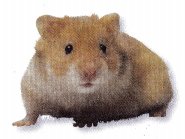(A) 10
(B) 12
(C) 15
Given,
There are five dogs, seven cats and three hamsters
By adding five with seven we get twelve and then by adding twelve with three we get the sum as fifteen,
So the answer is there are fifteen pets.

Question 5.
Children brought toys to school. 3 children brought balls. 6 children brought games. 8 children brought trucks. How many toys did the children bring to school?(A) 17
(B) 14
(C) 9
Given,
Three children brought balls,
Six children brought games,
Eight children brought trucks,
By adding three with six we get nine and then adding nine with eight we get seventeen as the sum,
So children brought seventeen toys to the school.

Question 6.
Without adding, circle the group of numbers that you think will have the greatest sum.